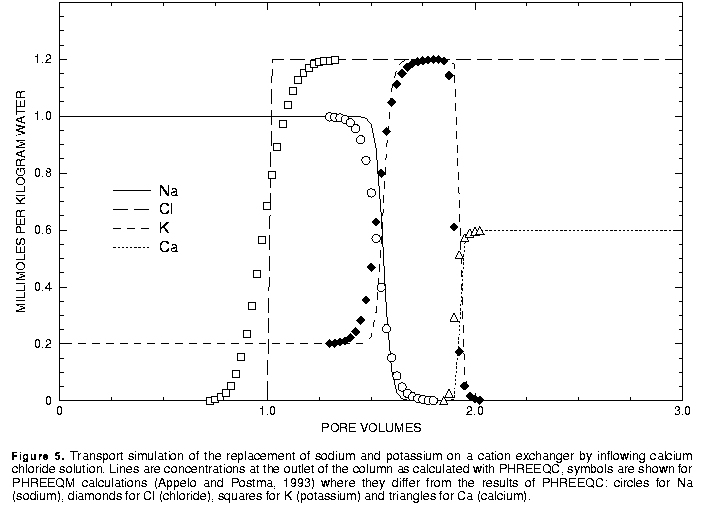[Next] [Previous] [Up] [Top]

EXAMPLES

## Example 9.-- Advective Transport and Cation Exchange

The following example of advective transport in the presence of a cation exchanger is derived from a sample calculation for the program PHREEQM (Appelo and Postma, 1993, example 10.13, p. 431-434). The chemical composition of the effluent from a column containing a cation exchanger is simulated. Initially the column contains a sodium-potassium nitrate solution in equilibrium with the cation exchanger. The column is then flushed with three pore volumes of calcium chloride solution. Calcium, potassium, and sodium react to equilibrium with the exchanger at all times. Dispersion is included in the calculations of Appelo and Postma, but PHREEQC lacks the capability to calculate dispersive effects.

The input data set is listed in table 17. The column has 40 cells to be consistent with one of the runs described by Appelo and Postma (1993). The solution filling each of the 40 cells of the column is defined with the SOLUTION 1-40 keyword data block. The infilling solution for the column must be defined as SOLUTION 0, and it is a calcium chloride solution. The amount and composition of the exchanger in each of the 40 cells is defined by the EXCHANGE 1-40 keyword data block. The number of exchange sites in each cell is 1.1 mmol, and the initial composition of the exchanger is calculated such that it is in equilibrium with solution 1. Note that the initial exchange composition is calculated assuming that the composition of solution 1 is fixed, that is, the composition of solution 1 is not changed during the initial exchange calculation.

### Table 17. Input data set for example 9

```TITLE Example 9.--Transport and ion exchange
SOLUTION 0  CaCl2
units   mmol/kgw
pH      7.0     charge
pe      8
temp    25.0
Ca      0.6
Cl      1.2
SOLUTION 1-40  Initial solution for column
units   mmol/kgw
pH      7.0     charge
pe      8
temp    25.0
Na      1.0
K       0.2
N(5)    1.2
EXCHANGE 1-40
equilibrate 1
X       0.0011
USE exchange none
TRANSPORT
-cells  40
-shifts 120
PRINT
-reset  false
SELECTED_OUTPUT
-file ex9.pun
-totals Na Cl K Ca
END
```
The number of cells to be used in the transport simulation and the number of times to shift the contents of each cell to the next cell are defined with the TRANSPORT keyword data block. In this example, 40 cells are used. This requires that 40 solutions, numbered 1 through 40, be defined; the number of the solution corresponds to the number of the cell in a column. In this example, all cells contain the same solution, but this is not required. Solutions could be defined differently for each cell and could be defined by reactions in the current or preceding simulations (using the SAVE keyword). The definition of a solution for each cell is mandatory, but the definition of an exchanger for each cell is optional. The number of the exchanger corresponds to the number of the cell in a column, and if an exchanger is defined for a cell number, then it is used in the calculations for that cell. In this example, an identical exchanger is defined for each cell.

The USE data block (table 17) is necessary to eliminate an implicitly defined reaction after the initial solution and initial exchange composition have been calculated. (Such a reaction step would not be an error, but the results would indicate no net reaction because the exchanger is already in equilibrium with last solution defined.) The PRINT keyword is used to eliminate all printing to the output file. The SELECTED_OUTPUT data block specifies that the total dissolved concentrations of sodium, chloride, potassium, and calcium will be written to the file ex9.pun. The selection of the master species for exchanger X occurs in the default database file in the EXCHANGE_MASTER_SPECIES data block; the exchange reactions are defined by the EXCHANGE_SPECIES data block of the default database file.

The results for example 9 are shown by the curves in figure 5. Also shown are the results of PHREEQM simulations for the same problem, except that dispersion was included in the PHREEQM calculations. Only the points from the PHREEQM calculations that differ from the PHREEQC results are included on figure 5. The main features of the calculations are the same between the two models. Chloride is a conservative solute and begins to be eluted at about one pore volume. The sodium initially present in the column, exchanges with the incoming calcium and is eluted until it is exhausted at about 1.5 pore volumes. Because potassium exchanges more strongly than sodium (larger log K in the exchange reaction), potassium is released after sodium. Finally, when all of the potassium has been released, the concentration of calcium has increased to a steady-state value equal to the concentration in the infilling solution.The differences between the two model simulations are due entirely to the inclusion of dispersion in the PHREEQM calculations. The breakthrough curve for chloride in the PHREEQM calculations coincides with an analytical solution to the advection dispersion equation for a conservative solute (Appelo and Postma, 1993, p. 433). Without dispersion, PHREEQC models the advection of chloride as a square-wave front of chloride concentration. The characteristic smearing effects of dispersion are absent in the fronts calculated for the other elements as well, although some curvature exits due to the effects of the exchange reactions.

Table 17. Input data set for example 9

User's Guide to PHREEQC - 07 MAY 96
[Next] [Previous] [Up] [Top]